### What is varactor diode?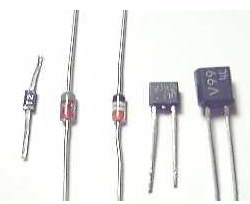Varactor diode

Varactor diode is named because of its variable capacitance property of the diode. The capacitance is varied by changing the width of the depletion region by controlling the reverse biased voltage.

It always works in reverse biased condition. The other names of varactor diode are varicap, voltcap, variable capacitance or tunning diode.

### Symbol of varactor diode:

The symbol consists of a capacitor at the end of the diode, which represents the variable capacitance of the diode.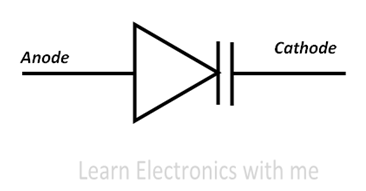Symbol of Varactor diode

### Construction of varactor diode: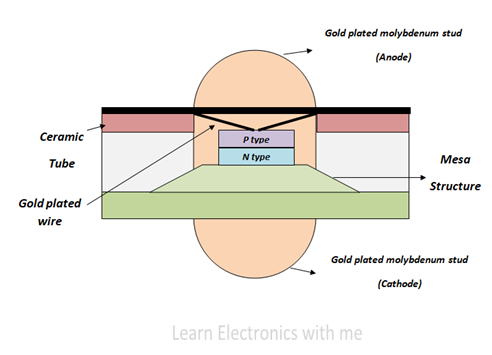Construction of Varactor diode

It consists of P type and N type semiconductor material which is made up of Silicon or Gallium arsenide depending upon the application. Silicon is used for low frequency applications and Gallium arsenide is used for high frequency applications.

These P type and N type material is placed on the Mesa table like structure. In the other type of diodes both the materials are uniformly doped but in varactor diode near the PN junction the concentration of impurity is less and gradually increases at the other side of the layer. The entire structure is covered by ceramic except some portion of Molybdenum stud.

### Working of varactor diode:

Before we see about the working of varactor diode, we should know about the capacitor.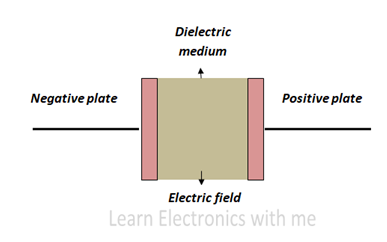Structure of Capacitor

It consists of two plates, one is positively charged and the other is negatively charged. These plates are separated by non conductive material which is called dielectric medium.

When the voltage is applied positive charges get accumulated on one side and negative charges at the other side. These accumulation of charge is called capacitance

When the size of the dielectric medium is less and when the charged plates are near, attractive force is more and more charges gets accumulated and thus it increases the capacitance. When the plates are moved away capacitance decreases.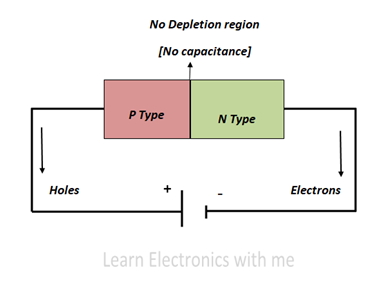Varactor diode when forward biased

When the varactor diode is forward biased the current starts to flow and thus the depletion region decreases.

When the voltage is increased further the depletion region completely disappears and charge cannot be stored.  Thus the varactor diode does not operate in forward biased condition.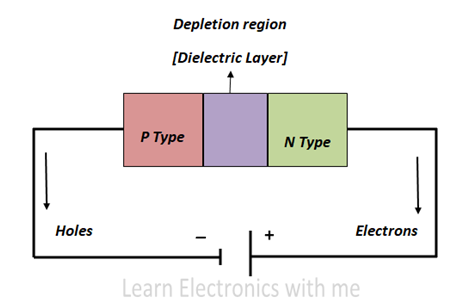Varactor diode when reverse biased

When the diode is reverse biased and the reverse biased voltage is increased the size of the depletion region increases. This depletion region acts like a insulator and prevents current to flow.

Respective charges gets accumulated along the junction forming some capacitance which is called junction capacitance. Varactor diode is designed in such a way to store these charges and thus it acts like the capacitor.

### V-I Characteristics of varactor diode: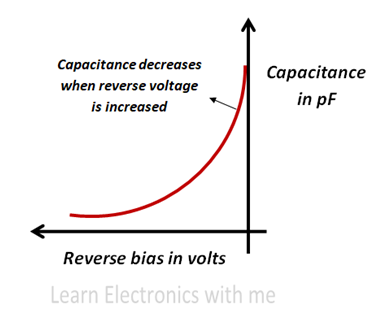V-I Characteristics of Varactor diode

The varactor diode has a non linear capacitance curve. We can see from the above figure that the capacitance is inversely proportional to the reverse bias voltage.

• It is smaller in size and light weight
• Produces less noise
• Power loss is very less
• Low price
• Portable